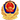矿山名称： 项目编号： 山阴县国土资源局山阴县燕庄村 整合建筑用砂矿采矿权挂牌出让公告 B1406000000000029 2018-02-09 山阴县国土资源局山阴县燕庄村 整合建筑用砂矿采矿权挂牌成交公示 山阴县 17.9 采矿权出让 0 山阴县强胜砂石有限公司 法人 911406215733552126 采购人 220 山阴县北周庄燕庄村 朔州市公共资源交易中心（朔州市政务大厅三楼） 2018-02-09 一次性缴纳 2018-02-09 位于山阴县325度方向直距15km，燕庄村北西 http://www.mlr.gov.cn/kyqsc/ 1. x=4389003.00；y=38389744.00 2. x=4389087.00；y=38389427.00 3. x=4389284.36；y=38389418.42 4. x=4389307.04；y=38389778.33 5. x=4389384.97；y=38389787.98 6. x=4389423.40；y=38389681.87 7. x=4389797.75；y=38389689.15 8. x=4389813.20；y=38389563.40 9. x=4390218.08；y=38389682.85 10x=4390218.08；y=38389843.87 11x=4390284.29；y=38389864.28 12x=4390293.35；y=38389705.06 13x=4390344.00；y=38389720.00 14x=4391016.00；y=38390188.00 15x=4390566.00；y=38390630.00 16x=4390348.88；y=38390534.24 17x=4390378.84；y=38390359.98 18x=4390292.46；y=38390338.58 19x=4390116.83；y=38390431.89 20x=4389514.00；y=38390166.00

 主办单位：朔州市公共资源交易中心 | 中文域名：朔州市公共资源交易中心.公益 地址：山西省朔州市开发区招远路8-3号市政务服务中心三楼 网站标识码1406000030  晋ICP 备07500137号晋公网安备14060202000030号 联系我们 |  网站地图 版本号 V1.0